Next: Examples of GRB Localizations Up: Directions of GRBs with Previous: The Monte Carlo Model   Contents

## The Direction Response Matrix

The local sky with respect to the BeppoSAX frame of reference (fig.) has been split according to a grid of 576 directions: 36 azimuthal steps in the range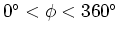and 16 polar steps in the range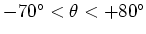; as for the photon energies, 11 logarithmic steps in the range 30-1000 keV have been chosen.

For each configuration obtained by combining each energy step with a single direction an MNCP simulation has been produced, using a monochromatic parallel beam of 5x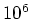photons; each single run has given the number of counts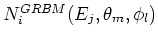detected by the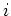th GRBM unit (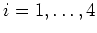), in the 40-700 keV (GRBM) band, with input photon energy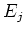() and incoming direction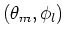(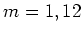;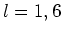). The energy grid has been refined to 1 keV steps by interpolating the measuredby means of cubic splines. When considering a GRB coming from the local direction, with a power law photon spectrum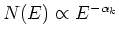, where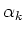is the power law photon index (which is a good description of real burst spectra at zero order approximation), the 40-700 keV counts in theth GRBM unit are expected to be the following: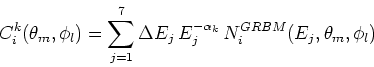(6)

The power law photon indexis assumed to take the following values: 1.0, 1.5, 2.0 (when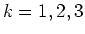, respectively): these values have been chosen in order to account for the range of typical photon indices of measured bursts. The best estimate of the burst incoming direction is assumed to minimize the following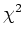statistics: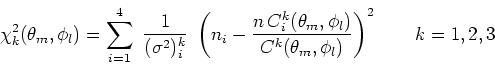(7)

where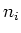expresses the GRB total counts in theth GRBM unit in the 40-700 keV (GRBM) channel;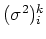is the variance ofplus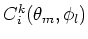;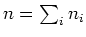; and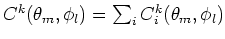.

In order to refine the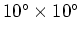angular grid, for anythe expected counts(eq.) have been interpolated with bicubic splines down to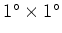cells. The best estimate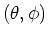for the GRB direction is given, according to the following procedure: once the(eq.) is computed, the solutions that are likely to be the burst direction are found in correspondence of the relative minima. The fact that there are often more than one candidate position is mainly connected with two factors: first, the geometrical symmetry of the GRBM configuration, only partially modified by the surrounding material; second, possible albedo effects due to the Earth atmosphere, that have not been taken into account yet.

Finally, another similar technique aimed to reconstruct the burst directions from the total counts left in each GRBM detector unit was developed by [Preger et al., 1999]: while theminimization technique was on principle the same, in that case, the GRBM response matrix was derived from the results of the on-ground calibration tests ([Amati, 1998]), performed with different radioactive sources at different directions with respect to the satellite frame of reference. In the majority of cases, the two methods have given consistent results in localizing the same bursts. For a complete review of the Monte Carlo model see [Calura et al., 2000b], while for the reconstruction method based on the on-ground calibrations, see [Preger et al., 1999b].Next: Examples of GRB Localizations Up: Directions of GRBs with Previous: The Monte Carlo Model   Contents
Cristiano Guidorzi 2003-07-31